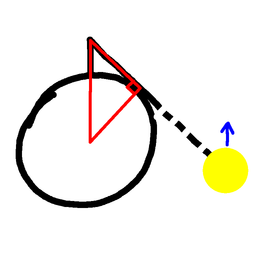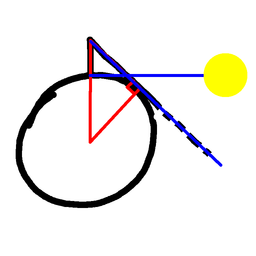# Radius Of The Earth Part TwoSubscribe!My latest posts can be found here:
Previous blog posts:

## 2017/09/16 - Radius of the Earth - Part 2

Some nine and a bit weeks ago I posted about a method of calculating (or estimating) The Radius Of The Earth using a stopwatch and watching the Sun at sunrise (or sunset). When "Mike the Sundial" told me the idea I was just stunned at the simplicity and elegance. Colin Beveridge took up the challenge, and you can read his account of his method here:

 In a moment you'll say "Hang on - you're supposed to be calculating the size of the Earth, but here you are assuming you know it!" What I'm doing here is working forward from the radius and calculating the time. Then we can reverse the calculation - if we know the time we can calculate the radius. So the initial calculation is just to set the scene, and familiarise ourselves with the calculations we'll be doing.
When I read that I was interested to see just how different his take was from mine, but then I realised - it comes down to the phenomenon of "chunking". ColinB was working - as most people would - from first principles.

But I have a piece of knowledge that ColinB doesn't have, and I hadn't realised just how much it affected my approach. I know the formula for the distance to the horizon.

Well, actually, I don't know the formula, but I do know that when you are 5 metres above sea level, the distance to the horizon is about 8000 metres. What's more, I know how to compute that value, so if I need to know it more accurately, I can just sketch the diagram and compute the answer.Before Sunrise
How does that help?

Consider the minute or two before sunrise. Let's suppose we are at the equator, and it's the equinox. Later we can correct for all these things, but it makes our initial calculations easier. So the picture here shows the moment before sunrise. The Sun is just illuminating the top of a 5 metre tall wall, and the sunlight is now descending the wall. We're timing how long it will take to get to the baseSunrise for the base of the wall
And now we see the situation as the sunlight reaches the base of the wall. The obvious thing to do is now consider the angle at the centre of the Earth, etc, but there is something else we can do. We know that the point of contact with the Earth of the Sun's rays has rolled over the Earth's surface, and we know that it's come from 8000 metres away. So the time it's taken the sunlight to descend our wall is the time taken for the tangent to roll 8000 metres around the Earth.

But we also know that the Earth's circumference is 40 million metres, because that's the original definition of the metre, so the distance that point of contact has moved is 8000/40,000,000 or $1/5000^{th}$ of the Earth's circumference. So the time taken is $1/5000^{th}$ of a day.

"Ah!" you say, "But you're assuming we know the size of the Earth!"

Yes, in all the above I've been assuming I know how big the Earth is, and I've calculated an approximation of the time taken for light to descend a 5 metre wall. But now we can go back and replace all the figures with letters and follow the calculation through. When we do that we get a relationship between the Earth's radius/circumference and the time taken to descend a wall. Instead of using 8000 we use $\sqrt{2.R.h}$ where $R$ is the Earth's radius, and $h$ is the height of the wall. Instead of 40 million metres we use $C,$ which is $2\pi{R}.$ So using $C$ as the Earth's circumference, $R=C/(2\pi),$ $D=\sqrt{2hR}=\sqrt{2hC/(2\pi)}=\sqrt{hC/\pi}.$

That means the distance the sunlight rolls as a fraction of a day is $D/C$ which comes out as $\left(\sqrt{hC/\pi}\right)/C = \sqrt{h/(\pi{C})}.$ For brevity we write $S=86400$, we get:

$T = S\sqrt{\frac{h}{\pi{C}}}$

We can turn that around, and we get:

$C = \left(\frac{S}{T}\right)^2 \frac{h}{\pi}$

We're on the final straight. We've computed the size given the time to descend from some height $h$ to the bottom of the wall, which is assumed to be at sea level. Suppose instead, as ColinB did, we go from some height $2h$ to the lower height of $h$ - what then?

We go back to the equation telling us $T$ from $h$ and $C.$ If we double $h$ then $T$ goes up by a factor of $\sqrt{2}.$ So that means the time from $2h$ to $h$ is $\sqrt{2}-1$ times the time from $h$ to 0. So take the time measured, divide by $\sqrt{2}-1,$ and use that.

So if $T$ is the time taken from $2h$ to $h$ then we use $\frac{T}{(\sqrt{2}-1)}$ and put that in the above formula. So now we get:

$C = \frac{S^2.h}{\pi{(T/(\sqrt{2}-1))^2}}$

which finally simplifies to:

$C = \left(\frac{S}{T}\right)^2\frac{(\sqrt{2}-1)^2}{\pi}h$

We've used $T$ as the time in seconds. If instead we use $t$ as the time in days then the $S$ disappears, and if we set $h$ to be 2 then we get:

$C = \frac{0.109}{t^2}$

## Correcting for latitude ...

At a latitude of $\theta$ the radius we measure is a factor of $cos(\theta)$ too small, so we need to divide by $cos(\theta)$. A latitude of 53.13 degrees corresponds to a correction of 0.6. So with $t$ in days we get:

$C = \frac{0.182}{t^2}$

## Sanity check ...

We know the Earth's circumference is 40 million metres, and plugging that in we get a time of 5.828 seconds for sunrise to descend from the 4 metre height to 2 metre height.

Better be quick!

 <<<< Prev <<<< Grep Timing Anomaly : >>>> Next >>>> The Four Points Puzzle ...You can follow me on Mathstodon.

 Of course, you can alsofollow me on twitter:## Send us a comment ...

 You can send us a message here. It doesn't get published, it just sends us an email, and is an easy way to ask any questions, or make any comments, without having to send a separate email. So just fill in the boxes and then

 Your name : Email : Message :

# ContentsSuggest a change ( <-- What does this mean?) / Send me email